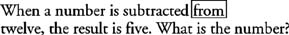## Keywords Indicating Equality

One of the hardest parts of solving word problems is creating the correct equation after reading the problem. Many people agree that, in comparison to setting up the correct equation, solving the equation is easy.

The following keywords indicate equality and, when translated, become the equal symbol, =.

• IS
• IS EQUAL TO
• EQUALS
• YIELDS
• RESULTS IN
• THE RESULT IS
• IS THE SAME AS

The direct translation strategy works for all the equality keywords, which means you do not need to change the order. The equal sign is placed exactly where the keyword is located.

Example 1: Translate the following sentence into an algebraic equation: Twice the difference between a number and five is equal to negative fourteen

Start by making the appropriate markings on the English sentence as you read it. Did you notice the adjacent keywords, the leading keywords, and the IS? Here's how you make the appropriate markings:

1. Put an open parenthesis between the adjacent keywords and close the parentheses before IS.

Twice (the difference between a number and five) is equal to negative fourteen.

2. Underline the two expressions to be subtracted as indicated by the leading keyword, DIFFERENCE BETWEEN.

Twice (the difference between a number and five) is equal to negative fourteen.

3. Use an arrow to remind you that AND is replaced by a minus sign.Note: The TO that is part of IS EQUAL TO is not a turnaround word. Remember that the direct translation strategy works for all the equality keywords. The expression on the left side of the equation does not have to be turned around with the expression on the right side.

4. Translate the equation.

The equation translates to 2( x − 5) = −14.

One of the most confusing keywords indicating equality is IS. In a translation problem, you may see more than one IS, as Example 2 demonstrates.

Example 2: Translate the following sentences into an algebraic equation: When a number is subtracted from twelve, the result is five.What is the number?

The second IS is the only one translated to the = symbol. Any IS placed directly in front of a keyword for an operation does not indicate equality. When a number directly follows IS, however, IS does indicate equality.

Remember: Mark the turnaround word to remind you to translate in the correct order.• The equation translates to 12 − x = 5.

Check more of your translation abilities with Example 3.

Example 3: Translate the following statement into an equation: The product of eight and a number yields twenty‐four.

What operation should replace AND in this equation? The leading keyword, PRODUCT OF, indicates the eight is multiplied by a number.Note: The first expression is translated, then the equal sign is translated, and then the expression on the right of the equal sign. Often, in mathematics, breaking the problem up into smaller pieces can increase your success. Instead of translating the whole equation at one time, translate the first expression, the equal sign, and then the second expression.